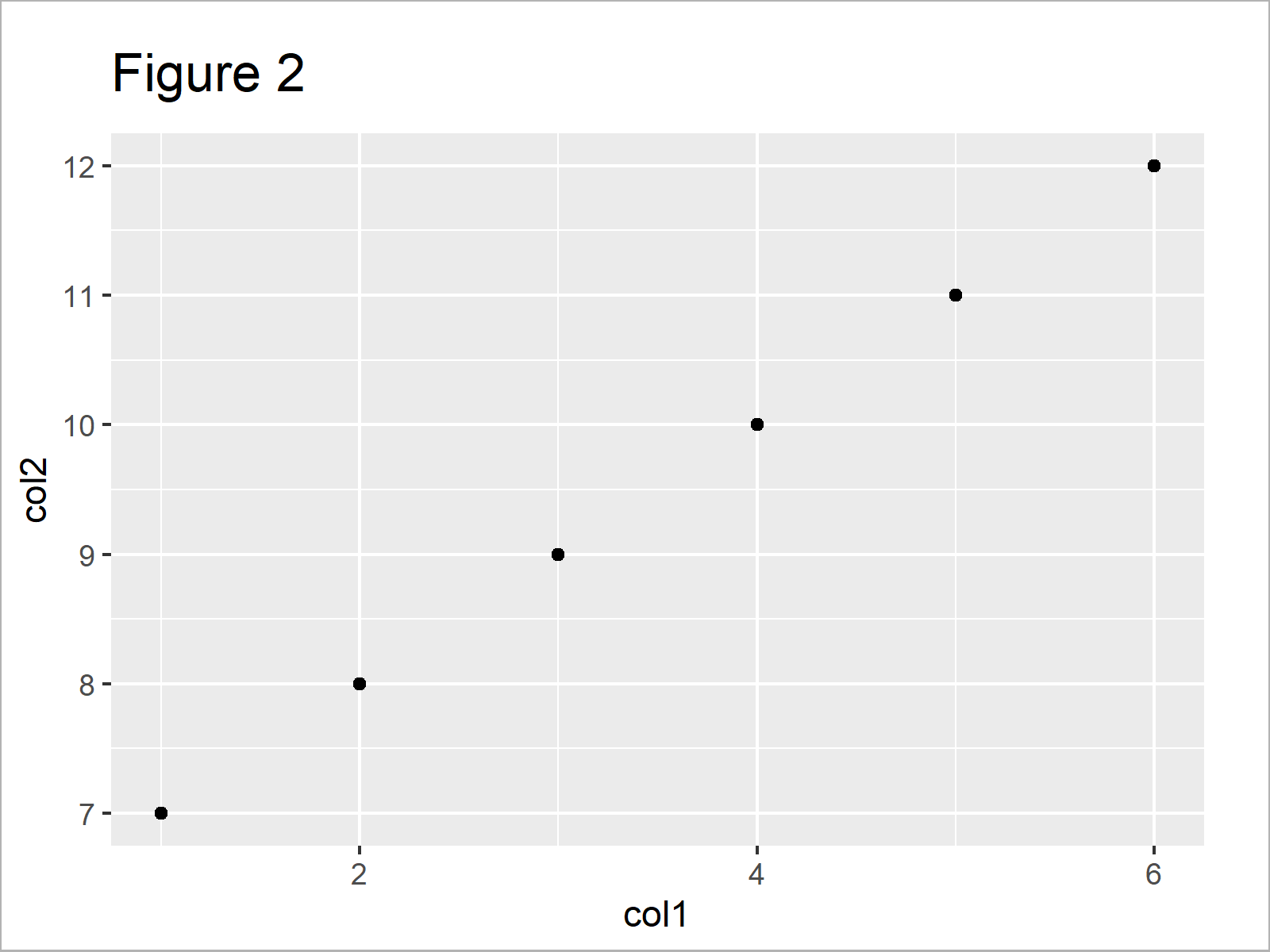# R ggplot2 Warning Message – Use of data\$X is discouraged. Use X instead

This tutorial explains how to avoid the ggplot2 warning message “Use of data\$column is discouraged. Use column instead” in R.

The page contains two examples for the handling of ggplot2 warnings. To be more specific, the content of the post looks as follows:

Let’s get started…

## Example Data & Add-On Packages

We’ll use the following data as basement for this R programming language tutorial:

```data <- data.frame(col1 = 1:6,    # Create example data
col2 = 7:12)
data                              # Print example data```Have a look at the table that got returned by the previous R programming code. It shows that our example data consists of six rows and the two integer columns “col1” and “col2”.

For the following tutorial, we’ll also have to install and load the ggplot2 package:

```install.packages("ggplot2")       # Install & load ggplot2 package
library("ggplot2")```

We have set up the examples. Let’s move on!

## Example 1: Reproduce the Warning Message – Use of data\$X is discouraged. Use X instead.

In this section, I’ll explain how to replicate the ggplot2 warning “Use of data\$column is discouraged. Use column instead” in the R programming language.

Have a look at the previous R syntax:

```ggplot(data,                      # Specify data within aes
aes(x = data\$col1,
y = data\$col2)) +
geom_point()
# Warning messages:
# 1: Use of `data\$col1` is discouraged. Use `col1` instead.
# 2: Use of `data\$col2` is discouraged. Use `col2` instead.```As shown in Figure 1, the previous syntax has plotted a ggplot2 scatterplot. However, you can also see that the RStudio console has returned the ggplot2 warning message “Use of data\$column is discouraged. Use column instead”.

The reason for this is that we have specified the name of our data frame within the aesthetics (i.e. the aes function) of our ggplot2 graphic.

So how can we avoid this problem?

## Example 2: Fix the Warning Message – Use of data\$X is discouraged. Use X instead.

Example 2 explains how to get rid of the ggplot2 warning message “Use of data\$column is discouraged. Use column instead”.

For this, we simply have to remove the name of our data frame within the aes function:

```ggplot(data,                      # Don't specify data within aes
aes(x = col1,
y = col2)) +
geom_point()```Figure 2 shows the output of the previous R syntax – We have created basically the same graph as in Example 1. However, this time the RStudio console didn’t return a warning message.

For clarification: Within the aes() function, we can simply use the names of our variables without specifying the data frame name in front of the variable name.

## Video & Further Resources

If you are interested in data visualization in R and the functions of the ggplot2 package, you have to watch the following video, where I explain the ggplot2 package in much more detail (beginners & advanced users).

Furthermore, you may have a look at the other tutorials on statisticsglobe.com. I have released several articles already:

You have learned in this tutorial how to deal with the ggplot2 warning message “Use of data\$column is discouraged. Use column instead” in the R programming language. If you have any additional questions, please let me know in the comments.

Subscribe to the Statistics Globe Newsletter